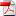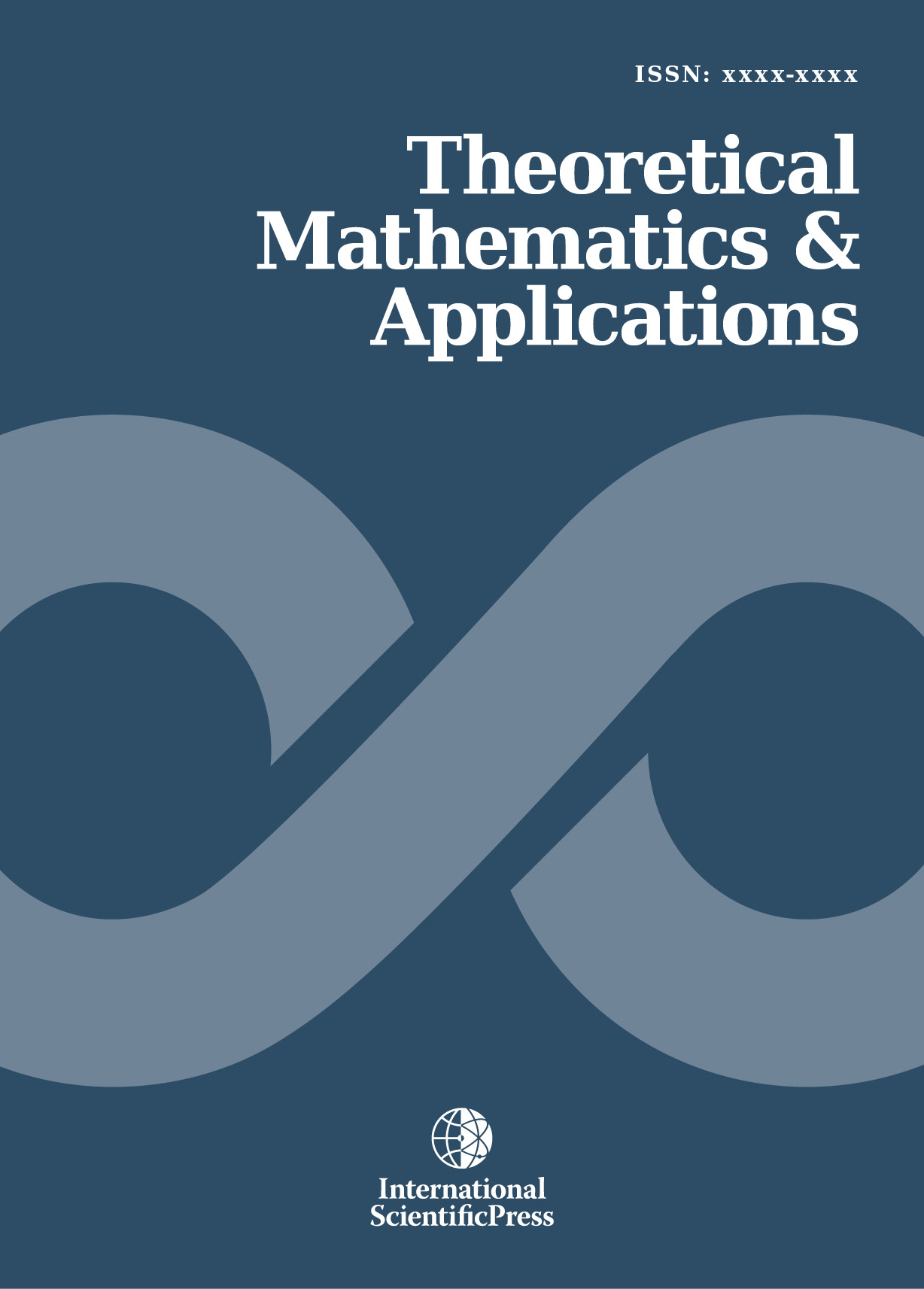# Theoretical Mathematics & Applications

#### Parametric euclidean algorithm

•[ Download ]
• Times downloaded: 2002
• Abstract

In this paper, we deal with the computation of generic greatest common divisors (gcd) of a finite set of parametric univariate polynomials. We will describe a parametric version of the well-known euclidean algorithm for computing gcds of univariate polynomials. We introduce the notion of parametric greatest common divisor in order to uniformly describe the gcd of univariate polynomials depending on parameters. The main algorithm of the paper decomposes the parameters space into a finite number of constructible sets such that a gcd of the parametric univariate polynomials is given uniformly in each constructible set.ISSN: 1792-9687 (Print)
1792-9709 (Online)